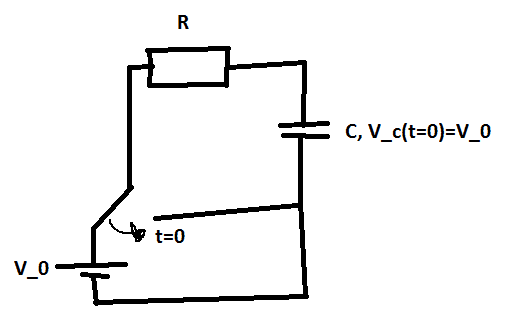# Deriving Capacitor Voltage for a Discharging Capacitor

Potatochip911

## Homework Statement

I'm trying to derive the voltage waveform across the capacitor for a discharging capacitor that has been fully charged by a DC power supply ##v_0##, i.e. ##v_c(t=0)=v_0## and then at ##t=0## the switch is flipped and the capacitor starts to discharge.## The Attempt at a Solution

From KVL we obtain
$$v_c=\frac{q}{c}=iR$$
Taking the time derivative of this and since capacitor is discharging ##-\frac{dq}{dt}=i##... $$-\frac{i}{RC}=\frac{di}{dt}\\ -\int_0^t \frac{dt}{RC}=\int_{i_0}^{i}\frac{di}{i}\\ -\frac{t}{RC}=\ln (\frac{i}{i_0})\\ i=i_0e^{-\frac{t}{RC}}$$
Now to solve for ##i_0## at t=0 ##\frac{q}{c}=i_0Re^{-\frac{t}{RC}}## becomes $$\frac{v_0}{c}=i_0R\\ i_0=\frac{v_0}{RC}\Longrightarrow i=\frac{v_0}{RC}e^{-\frac{t}{RC}}$$
Finally from KVL $$v_c=iR=\frac{v_0}{C}e^{-\frac{t}{RC}}$$

which is not the correct answer.

Homework Helper
Gold Member
vc=iR=v0Ce−tRC
which is not the correct answer
Of course it isn't. You can see it is dimensionally incorrect.(Vo/C doesn't give voltage).
v0c=i0R
Where does this come from?

•Potatochip911
Potatochip911
Of course it isn't. You can see it is dimensionally incorrect.(Vo/C doesn't give voltage).

Where does this come from?

I got that from the KVL equation (q/c=ir) and at t=0 so ##\frac{q(0)}{c}=i(0)R##

Homework Helper
Q/C=Vo, not Vo/C .

•Potatochip911
Potatochip911
Q/C=Vo, not Vo/C .
Whoops, after making the proper substitution then we get ##i_0=\frac{v_0}{R}\Longrightarrow i=\frac{v_0}{R}e^{-\frac{t}{RC}}\Longrightarrow V_c=iR=v_0e^{-\frac{t}{RC}}## which is the correct expression :)

Homework Helper
It is useful to check the dimensions during a derivation.•Potatochip911